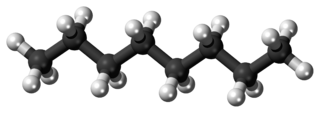# Coefficients Party, Part 8

Chemistry Level 1When liquid octane $(\ce{C_8H_{18}})$ combusts, it forms carbon dioxide and water vapor. The reaction can be modeled by the following, where $\alpha,$ $\beta,$ $\gamma,$ and $\delta$ are positive coprime integers, $\alpha\ \ce{ C_8{H}_{18}}(l)+\beta\ \ce{ O_2}(g)\rightarrow \gamma \ \ce{ CO_2}(g)+\delta\ \ce{ H_2O}(g)$ Find $\alpha+\beta+\gamma+\delta.$

×